# Arccos free online calculator

Arccos(x) calculator. Inverse cosine calculator.

Enter value of x (between -1 and 1) and hit "Calculate" button

°

Cosine calculator »

Arccos(x), cos-1(x), inverse cosine function.

## Arccos definition

The arccosine of x is defined as the inverse cosine function of x when -1≤x≤1.

When the cosine of y is equal to x:

cos y = x

Then the arccosine of x is equal to the inverse cosine function of x, which is equal to y:

arccos x = cos-1 x = y

(Here cos-1 x means the inverse cosine and does not mean cosine to the power of -1).

#### Example

arccos 1 = cos-1 1 = 0 rad = 0°

## Graph of arccos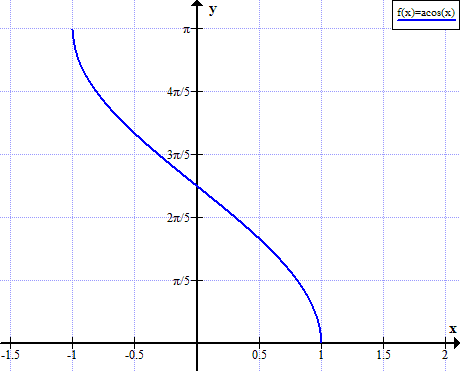## Arccos rules

Rule nameRule
Cosine of arccosinecos( arccos x ) = x
Arccosine of cosinearccos( cos x ) = + 2kπ, when k∈ℤ (k is integer)
Arccos of negative argumentarccos(-x) = π - arccos x = 180° - arccos x
Complementary anglesarccos x = π/2 - arcsin x = 90° - arcsin x
Arccos sumarccos(α) + arccos(β) =
arccos( αβ - (1-α2)(1-β2) )
Arccos differencearccos(α) - arccos(β) =
arccos( αβ + (1-α2)(1-β2) )
Arccos of sin of xarccos( sin x ) =  -x - (2k+0.5)π
Sine of arccosine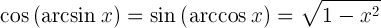Tangent of arccosine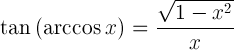Derivative of arccosine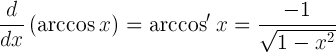Indefinite integral of arccosine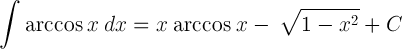## Arccos table

xarccos(x)

arccos(x)

(°)

-1π180°
-√3/25π/6150°
-√2/23π/4135°
-1/22π/3120°
0π/290°
1/2π/360°
2/2π/445°
3/2π/630°
10

Currently, we have around 1975 calculators, conversion tables and usefull online tools and software features for students, teaching and teachers, designers and simply for everyone.